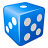Data Management on Hosted Feature Layer using Python API

907
3
06-16-2021 11:10 AMRegular Contributor

We are working with an existing Hosted Feature Layer in ArcGIS Online. The Hosted Feature Layer was created from a geodatabase and contains a number of feature classes and tables. 4 of the tables contain a a common field (let's call it "myField") that we want to update using the ArcGIS API for Python to calculate the fields. Drawing from my experience from arcpy, I would do something like:

1. Access the geodatabase
2. List out the tables
3. Iterate over the fields in those tables to find the tables that contains a field called "myField"
4. Run a calculate field function to calculate those fields based off of some logic.

So, in the API I have this so far to access my account and to grab an item (hosted feature layer) and calculate:

from arcgis.gis import GIS
import arcpy

hfl = gis.content.get(itemid)

hfl.calculate(where="OBJECTID = 1", calc_expression={"field": "myField", "value" : 1})

So, that worked on a hosted feature layer that contains only one table. As mentioned, mine has a number of tables so I need to grab the specific table(s) and calculate the "myField" field(s).

So, I tried to list out the tables of the hfl (hosted feature layer):

for t in hfl.tables:
print(t)

Which will return:

So, that function returns the URL's for the tables inside of the Hosted Feature Layer by index. I tried running the calculate on those tables, so I thought I could do something like:

for t in hfl.tables:
print(t)
t.calculate(where="OBJECTID = 1", calc_expression={"field": "myField", "value" : 1})

Which seems to run fine without any errors, but it doesn't calculate the field.

This is where I am at. I'm not convinced the way I access the tables in order to calculate the fields is correct. This is where I need some help.

As a side note, what I would also like to do (if possible) is grab some more properties of each of those tables. Like maybe access the table name or table index? Something similar to arcpy's method:

for t in arcpy.ListTables():
print(t.name)
if t.name == 'myTableName':
#do something

I'd like to also list out the fields of each table by name, similar to arcpy's method:

for f in arcpy.ListFields():
print f.name
if f.name == 'myField':
arcpy.CalculateField(#some calculate logic)

I'm looking to do much of the same rudimentary data management one can do on a geodatabase containing tables and fields one would do in arcpy. (i.e. list feature classes, list tables, list files, list fields, etc, etc)

The help document for the ArcGIS API for Python is quite lacking in both explanations and code examples.

Tags (5)
1 Solution

Accepted SolutionsbyEsri Contributor

Hi @MikeMacRae,

To update field values of a hosted feature layer's original table, you can use feature sets and spatial dataframe of the feature layer as follows:

# updating feature layer's attribute table
hfl = gis.content.get("feature layer item id")
hflayer =hfl.layers
#for multiple rows
fset = hflayer.query()
for f in (fset.features):
f.attributes['myfield'] = 'any value'
or
#for a single specific row
fset = hflayer.query(where="OBJECTID = 1")
fset.features.attributes['myfield'] = 'any value'

to update the joined tables of a feature layer, you can use the following script:

for table in hfl.tables:
if table.properties.name == "myTableName":
table.calculate(where="OBJECTID = 1", calc_expression={"field": "myField", "value" : 1})

to access the fields of a table:

for table in hfl.tables:
for field in table.properties.fields:
print(field.name)

I hope these give you more insights and are helpful.

======================================================================

3 RepliesbyEsri Contributor

Hi @MikeMacRae,

To update field values of a hosted feature layer's original table, you can use feature sets and spatial dataframe of the feature layer as follows:

# updating feature layer's attribute table
hfl = gis.content.get("feature layer item id")
hflayer =hfl.layers
#for multiple rows
fset = hflayer.query()
for f in (fset.features):
f.attributes['myfield'] = 'any value'
or
#for a single specific row
fset = hflayer.query(where="OBJECTID = 1")
fset.features.attributes['myfield'] = 'any value'

to update the joined tables of a feature layer, you can use the following script:

for table in hfl.tables:
if table.properties.name == "myTableName":
table.calculate(where="OBJECTID = 1", calc_expression={"field": "myField", "value" : 1})

to access the fields of a table:

for table in hfl.tables:
for field in table.properties.fields:
print(field.name)

I hope these give you more insights and are helpful.

======================================================================by